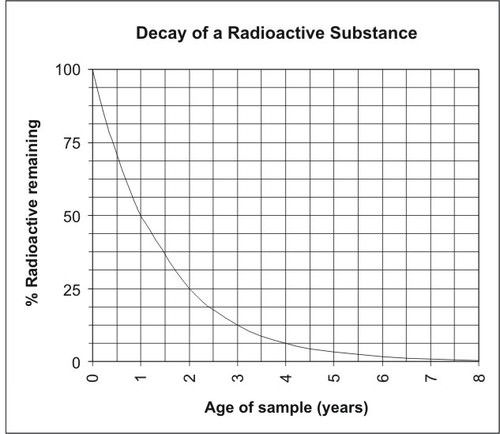# Evidence About Earth’s Past (Book)

## ABSOLUTE AGES OF ROCKS

### - Decay of Unstable Isotopes

Like other unstable isotopes, carbon-14 breaks down, or decays. For carbon-14 decay, each carbon-14 atom loses an alpha particle. It changes to a stable atom of nitrogen-14. This is illustrated in FigureUnstable isotopes, such as carbon-14, decay by losing atomic particles. They form different, stable elements when they decay. In this case, the daughter is nitrogen-14.

The decay of an unstable isotope to a stable element occurs at a constant rate. This rate is different for each isotope pair. The decay rate is measured in a unit called the half-life. The half-life is the time it takes for half of a given amount of an isotope to decay. For example, the half-life of carbon-14 is 5730 years. Imagine that you start out with 100 grams of carbon-14. In 5730 years, half of it decays. This leaves 50 grams of carbon-14. Over the next 5730 years, half of the remaining amount will decay. Now there are 25 grams of carbon-14. How many grams will there be in another 5730 years? Figure graphs the rate of decay of carbon-14.The rate of decay of carbon-14 is stable over time.